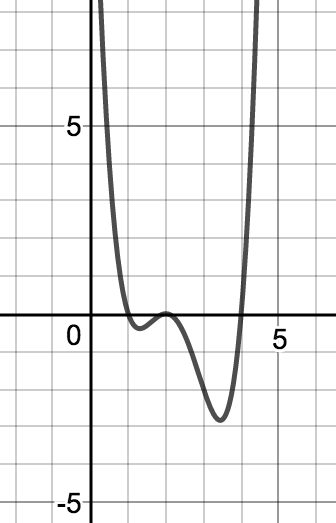# Polynomials

## Objective

Multiply polynomials, identifying factors, degrees, and number of real roots. Identify features of a polynomial in standard form.

## Common Core Standards

### Core Standards

?

• A.APR.A.1 — Understand that polynomials form a system analogous to the integers, namely, they are closed under the operations of addition, subtraction, and multiplication; add, subtract, and multiply polynomials.

• F.BF.B.3 — Identify the effect on the graph of replacing f(x) by f(x) + k, k f(x), f(kx), and f(x + k) for specific values of k (both positive and negative); find the value of k given the graphs. Experiment with cases and illustrate an explanation of the effects on the graph using technology. Include recognizing even and odd functions from their graphs and algebraic expressions for them.

?

• A.REI.A.1

## Criteria for Success

?

1. Use the understanding that multiplying polynomials follows the same principle as distribution to accurately find the product of polynomials.
2. Identify the resultant degree of a product of polynomials before calculating.
3. Identify the polynomials that are being multiplied as factors of the resultant polynomial.
4. Make conjectures about the end behavior of the polynomials based on the degree of the functions that are factors.

## Tips for Teachers

?

There are several strategies for multiplying polynomials. Ensure that students understand that all methods are ways to organize distribution of each term in one polynomial over all terms in the other polynomial.

## Anchor Problems

?

### Problem 1

What is the product of these pairs of polynomials written in standard form?

${ (x-1)(3x^2-2x+5)}$

${(x^2+y^2 )(x^3-2x+4)}$

### Problem 2

Explain how ${f(x)∙g(x)}$ results in the function ${ {h(x)}}$, both algebraically and graphically.

${f(x)=(x-2)^2}$

${g(x)=x^2-5x+4}$

${h(x)}$## Problem Set

?

The following resources include problems and activities aligned to the objective of the lesson that can be used to create your own problem set.

Find the product of ${(x-1)(x^3+4x^2+4x-1)}$